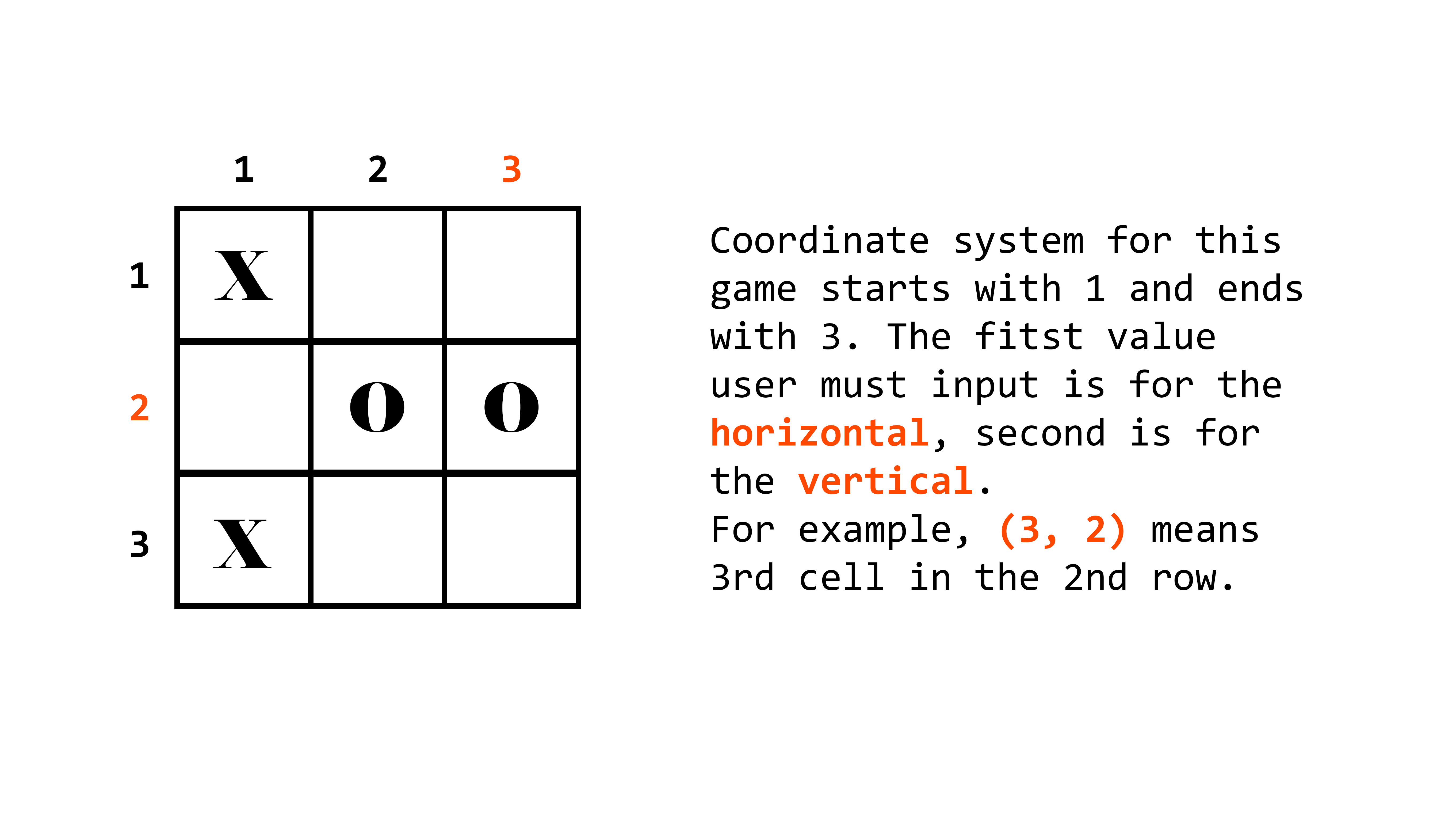Learn to Code via Tutorials on Repl.it!

← Back to all posts
Noughts & Crosses Game in 69 lines of Python code

It seems like many people enjoy tutorials about making games. Well, let me make one too! Today we are going to be implementing the legendary "Noughts and Crosses" game in 69 lines of Python code! I am not going to overcomplicate matters with OOP and all that... a few functions will do.

First, let's create 2 global variables:

``````# GLOBAL VARIABLES
M = [
['_','_','_'],
['_','_','_'],
['_','_','_']
]

S = True
C = 0``````

`M` stores our game's 3 x 3 field. Classic. It is a 2D matrix, so I called it `M`. `S` holds the side: when `S = True`, it's X's turn and vice versa. `C` holds the number of moves made.

Now, let's write the main function. I called it `xo()` for simplicity. It has to:

1. Show an empty board
2. Start a `while` loop that will repeat itself until `C == 9`
3. Inside the loop, it has to
1. Ask user to make a move
2. Check if either of the two players won and if yes, `break` itself
4. If the `while` loop doesn't encounter a `break` on its way, it means that the 9 moves are made, yet no one won, so it must be a tie!
``````# MAIN
def xo():
board_show()        # FUNCTION NOT YET IMPLEMENTED!
while C < 9:
turn()          # FUNCTION NOT YET IMPLEMENTED!
if big_check(): # FUNCTION NOT YET IMPLEMENTED!
print(f"{'X' if not S else 'O'} wins!", end="\n\n")
break
else:   # if break not encountered, it must be a tie!
print("It's a tie!", end="\n\n")``````

The easiest function to implement is `board_show()`. Just print out our little matrix `M`. Don't forget to put some empty `print`s for spacing purposes!

``````def board_show():
print()
for y in range(3):
print(" ", end="")
for x in range(3):
print(M[y][x], end=" ")
print()
print()``````

Now, the `turn()` function is a bit trickier, yet not too complicated either. We want to:

1. Ask player where he/she wants to place his/her mark
2. Check if the selected square is free, and if it is, put X or O into it, change the `S`tate, and `board_show()` to demonstrate the result
3. If it's not empty, we want to let the user know that the move was invalid and make him repeat it
``````def turn():
global S, C    # allows us to reference S that is not assigned in this scope
pos = [ ( int(i) - 1 ) for i in input("Your move: ").split() ]
# this produces a list of two int values X and Y
# it reduces each one by 1 since computer (unlike human) starts counting from 0
x, y = pos, pos   # save x and y separately for clearance
if M[y][x] == '_':
M[y][x] = 'X' if S else 'O'
S = not S
C += 1	# increment move counter C by 1 only if the move is valid
board_show()
else:
print("Invalid move!")``````Now that we have our `turn()` function nice and shining, we only need the `big_check()` function, only it's not so easy. The `big_check()` isn't called big for no reason -- it consists of three other functions:

1. `check_hr(y)` check `y`th row
2. `check_vr(x)` check `x`th column
3. `check_dig()` check both diagonals

But these are fairly simple and you will see why in a second. The only thing we need to do is to check whether all three positions have the same `chr` in them that is not `'_'` the default one.

``````def check_hr(y):
return M[y] == M[y] == M[y] != '_'

def check_vr(x):
return M[x] == M[x] == M[x] != '_'

def check_dig():
return M == M == M != '_' or \
M == M == M != '_'``````

Now, let's get to the `big_check()`. What it does essentially is it checks every row, column, and diagonal using the functions we've just written and if at least one of those function returns `True`, the whole `big_check()` function must return `True`!

``````def big_check():
win = False
for i in range(3):
if check_hr(i) or check_vr(i):
win = True
if check_dig():
win = True
return win``````

"Now we have everything! It's complete!" -- a newbie would say, but no, it's actually not. There is one last bit to it that will finally make it work -- the 69th line:

``xo()    # invoke the xo() function``